Open in App
Not now

# MongoDB – Comparison Query Operators

• Last Updated : 10 May, 2020

MongoDB uses various comparison query operators to compare the values of the documents. The following table contains the comparison query operators:

OperatorsDescription
\$eqIt is used to match the values of the fields that are equal to a specified value.
\$neIt is used to match all values of the field that are not equal to a specified value.
\$gtIt is used to match values of the fields that are greater than a specified value.
\$gteIt is used to match values of the fields that are greater than equal to the specified value.
\$ltIt is used to match values of the fields that are less than a specified valueo
\$lteIt is used to match values of the fields that are less than equals to the specified value
\$inIt is used to match any of the values specified in an array.
\$ninIt is used to match none of the values specified in an array.

In the following examples, we are working with:

Database: GeeksforGeeks
Collection: contributor
Document: three documents that contain the details of the contributors in the form of field-value pairs.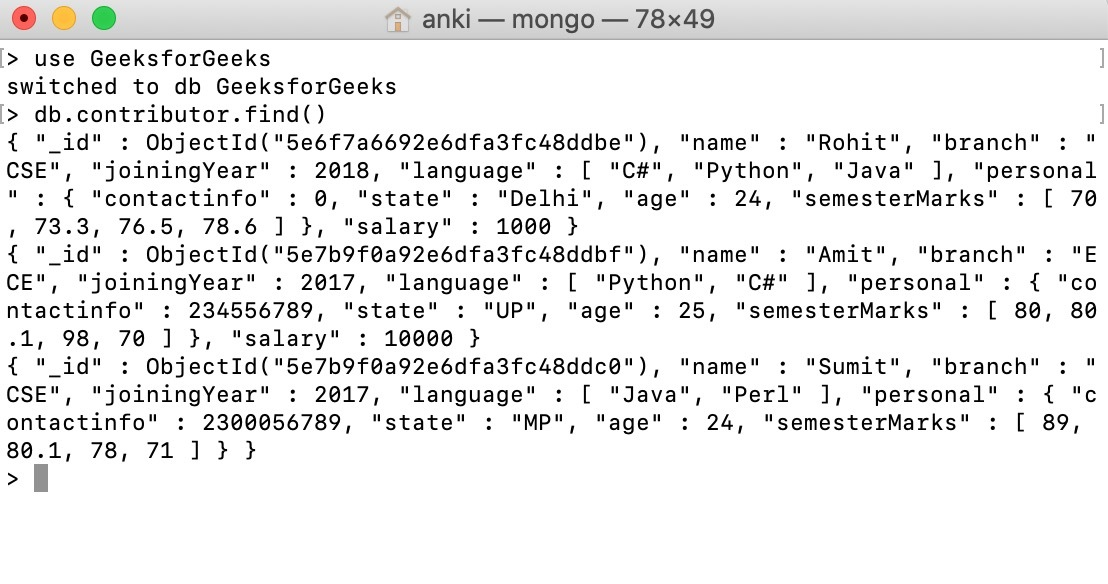## Matching values using `\$nin` operator:

In this example, we are retrieving only those employee’s documents whose name is not Amit or Suman.

 `db.contributor.find({name: {\$nin: [``"Amit"``, ``"Suman"``]}}).pretty()`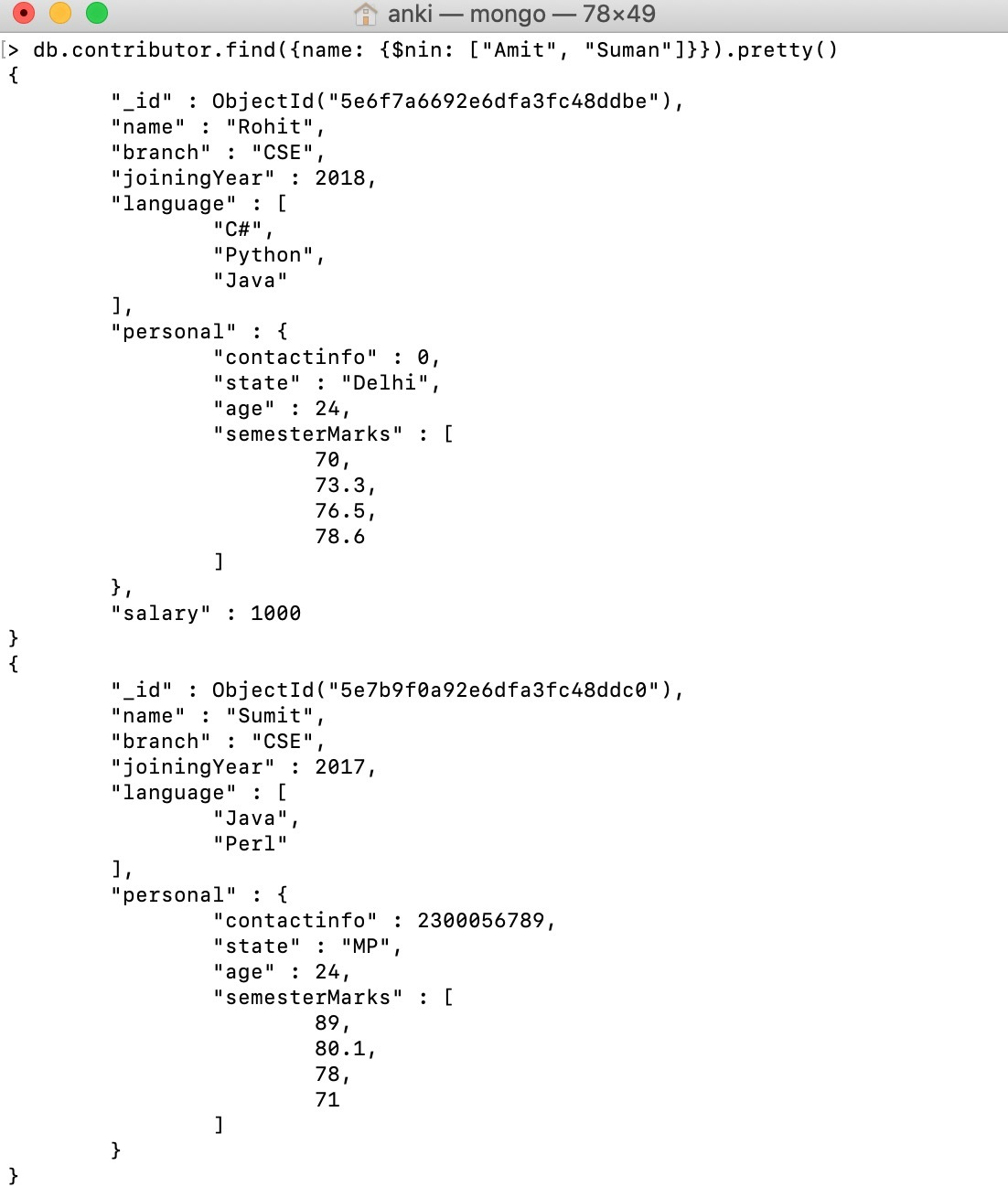## Matching values using `\$in` operator:

In this example, we are retrieving only those employee’s documents whose name is either Amit or Suman.

 `db.contributor.find({name: {\$``in``: [``"Amit"``, ``"Suman"``]}}).pretty()`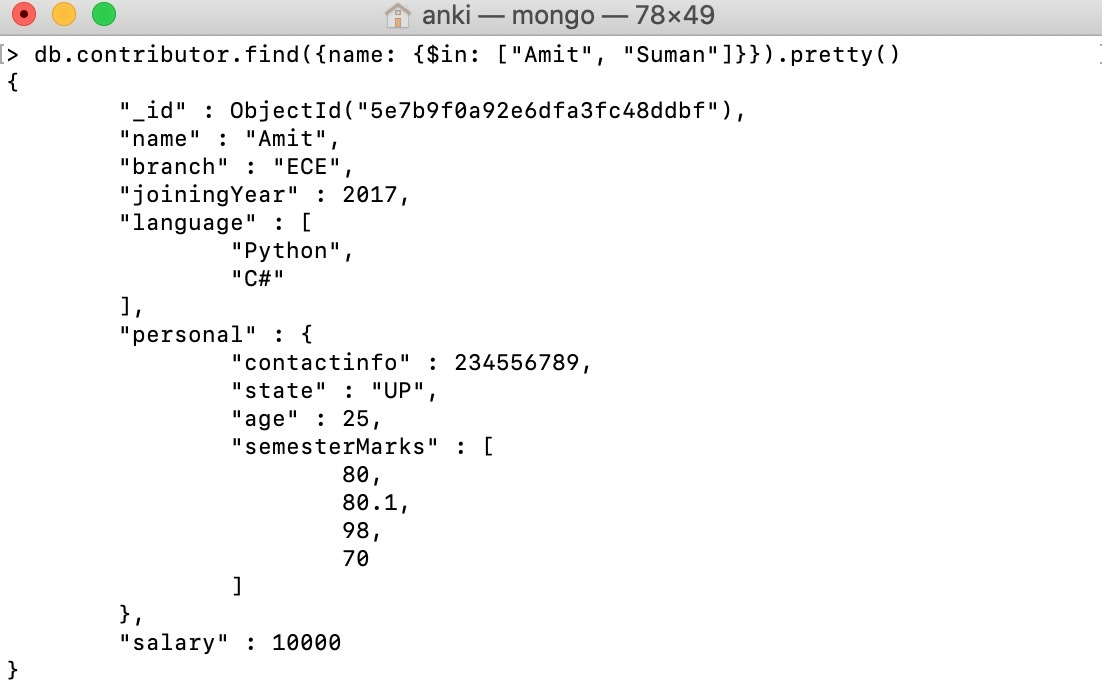## Matching values using `\$lt` operator:

In this example, we are selecting those documents where the value of the salary field is less than 2000.

 `db.contributor.find({salary: {\$lt: ``2000``}}).pretty()`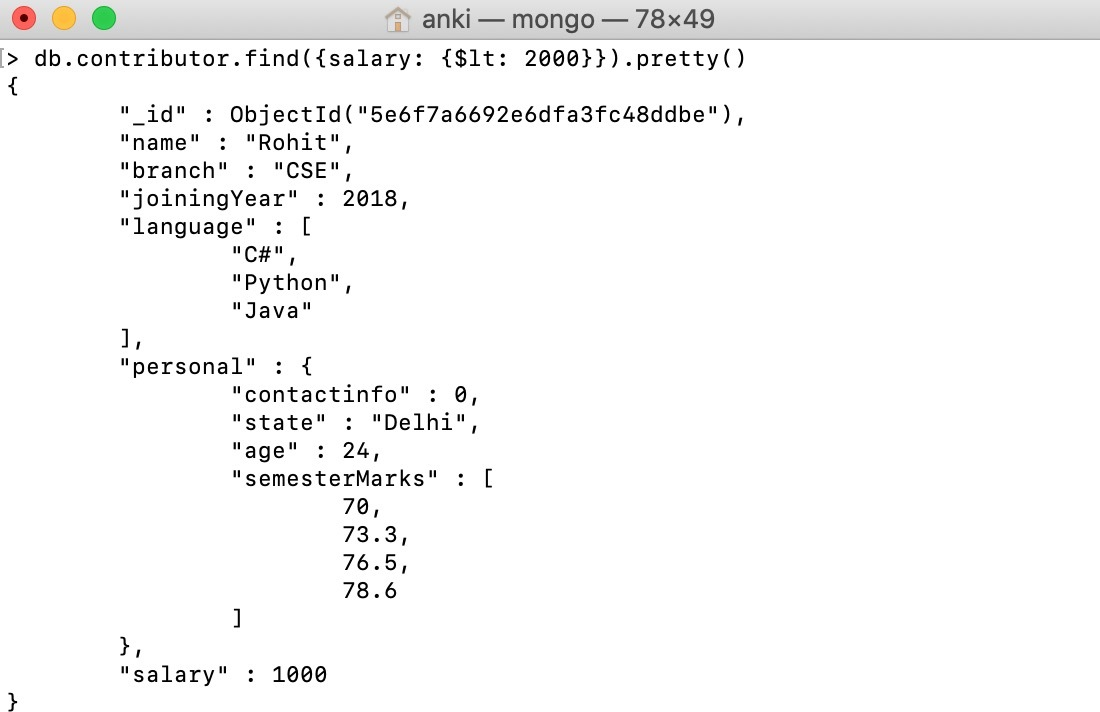## Matching values using `\$eq` operator:

In this example, we are selecting those documents where the value of the branch field is equal to CSE.

 `db.contributor.find({branch: {\$eq: ``"CSE"``}}).pretty()`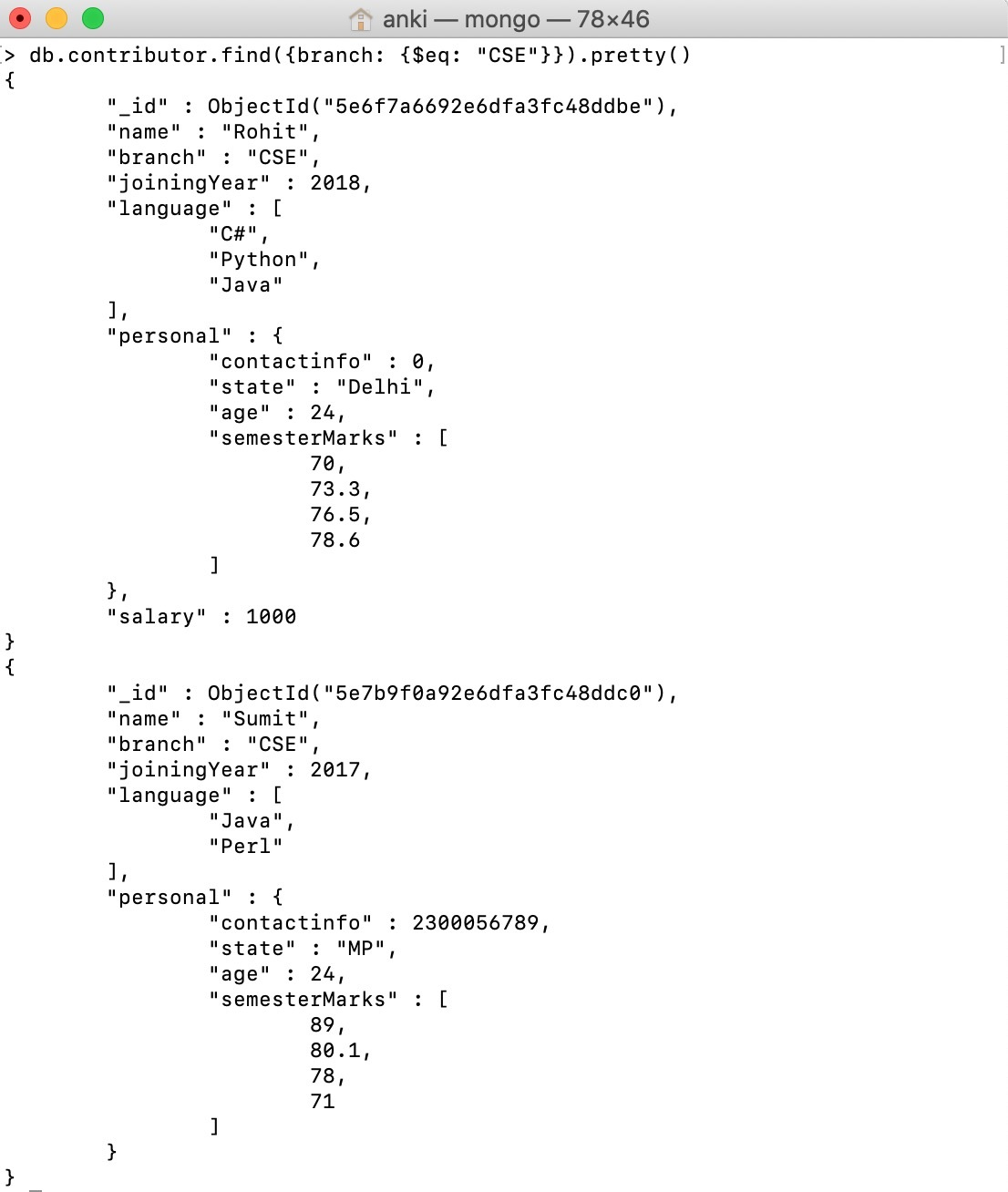## Matching values using` \$ne` operator:

In this example, we are selecting those documents where the value of the branch field is not equal to CSE.

 `db.contributor.find({branch: {\$ne: ``"CSE"``}}).pretty()`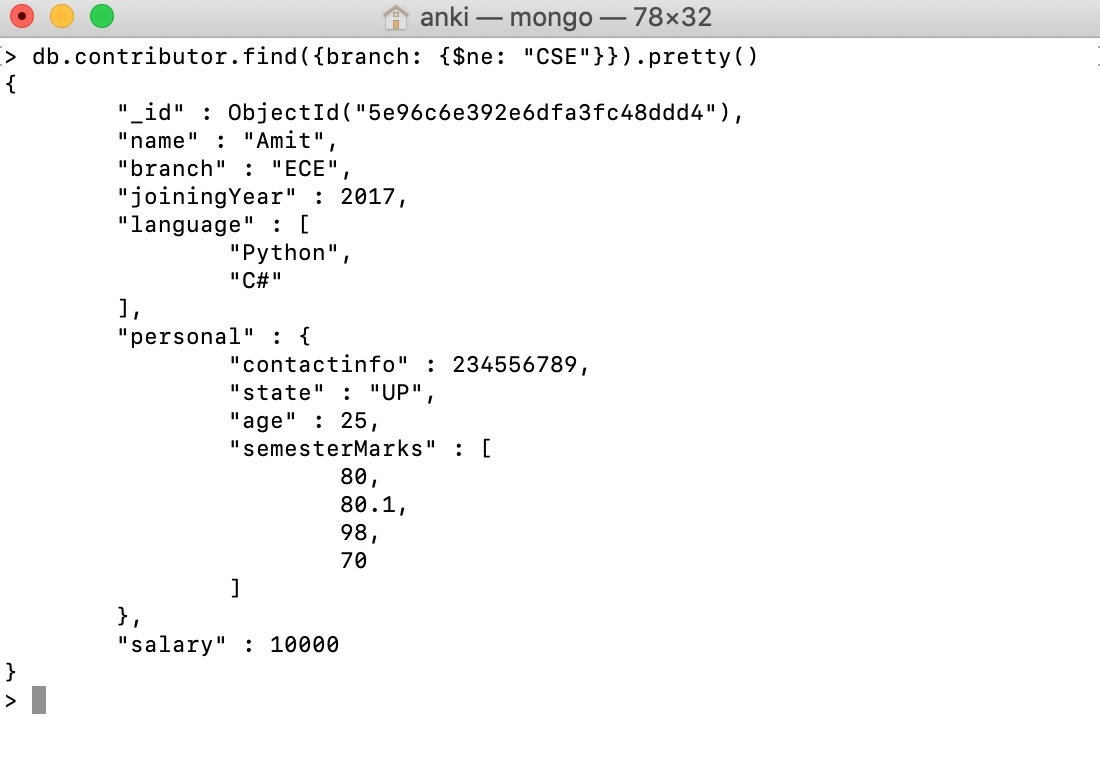## Matching values using `\$gt` operator:

In this example, we are selecting those documents where the value of the salary field is greater than 1000.

 `db.contributor.find({salary: {\$gt: ``1000``}}).pretty()`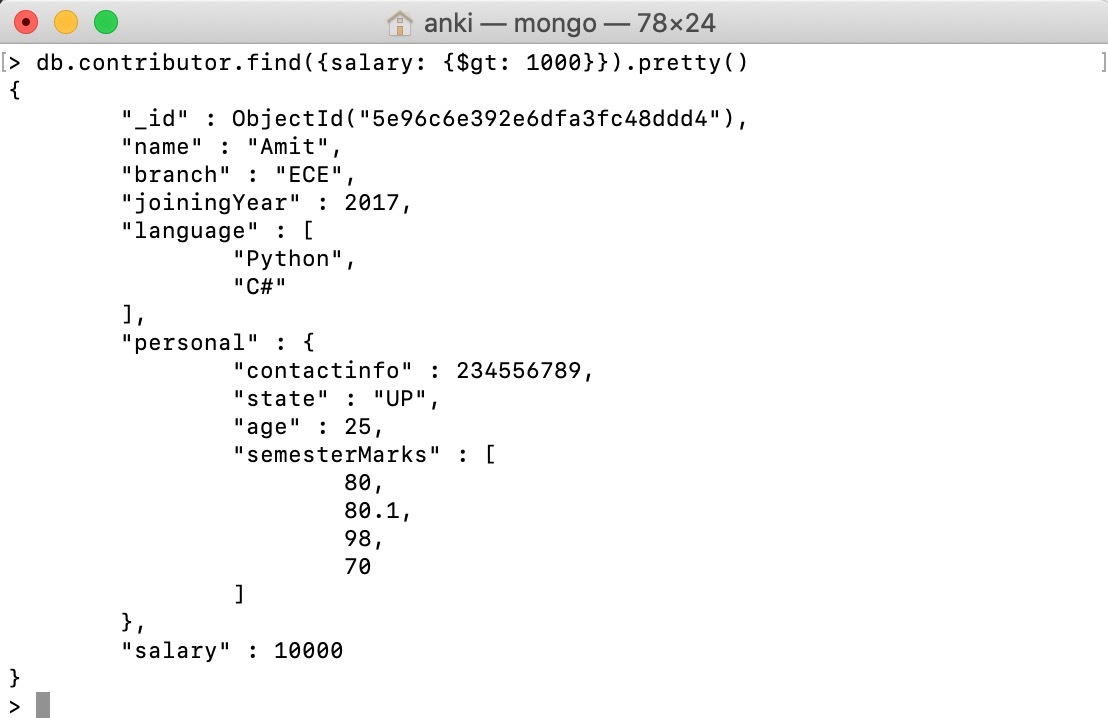## Matching values using `\$gte` operator:

In this example, we are selecting those documents where the value of the joiningYear field is greater than equals to 2017.

 `db.contributor.find({joiningYear: {\$gte: ``2017``}})`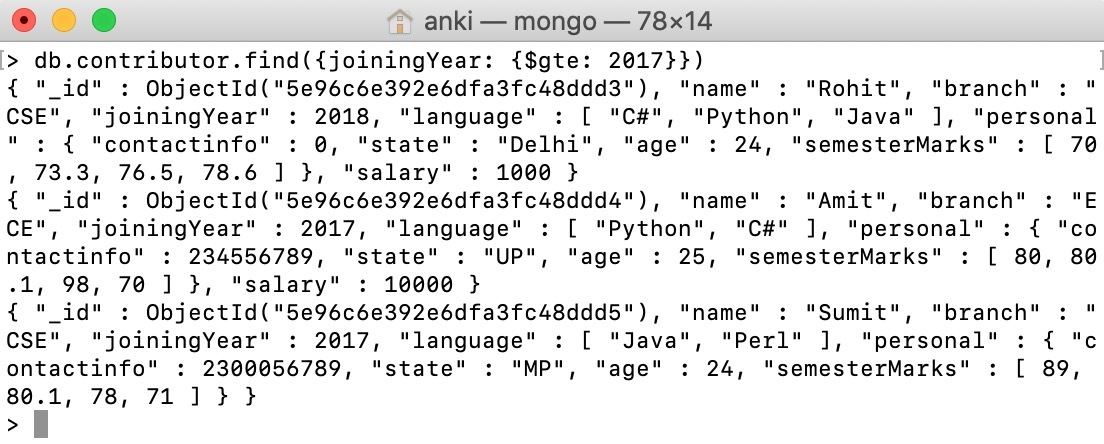## Matching values using `\$lte `operator:

In this example, we are selecting those documents where the value of the salary field is less than equals to 1000.

 `db.contributor.find({salary: {\$lte: ``1000``}}).pretty()`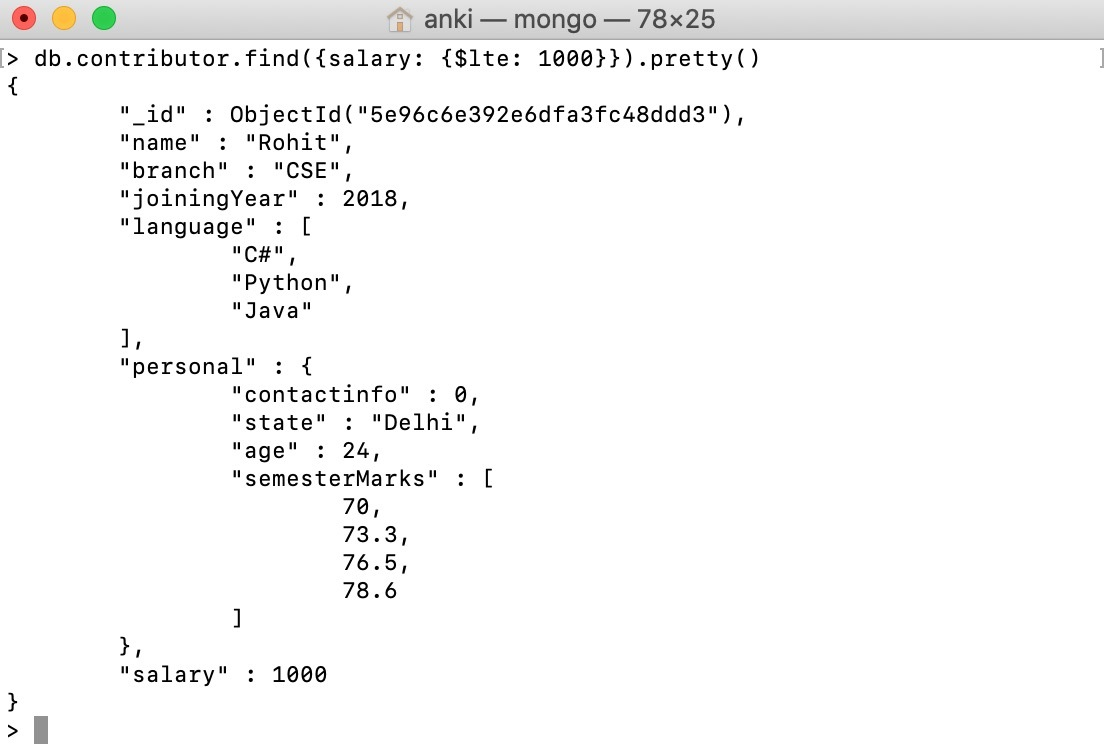My Personal Notes arrow_drop_up